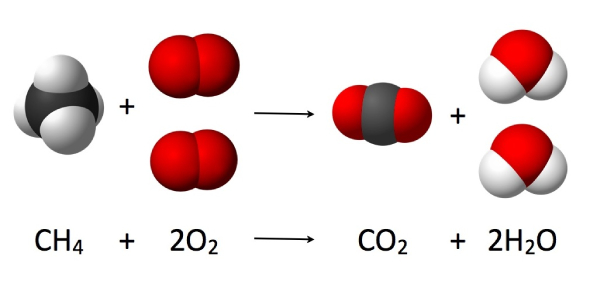# Stoichiometry Trivia Test: MCQ Quiz!

9 Questions | Total Attempts: 336Settings.

• 1.
Ammonia, , and copper (II) oxide react to form solid copper, water and nitrogen gas. What are the coefficients for the balanced chemical equations.
• A.

2,2,2,1,1

• B.

1,2,1,2,1

• C.

2,3,3,3,1

• 2.
If 40g of ammonia reacts with 80.0g copper (II) oxide, determine the limiting reactant. Use nitrogen gas as your unknown.
• A.

Ammonia

• B.

Copper(II) oxide

• 3.
Determine the mass of nitrogen gas produced in the reaction.
• A.

9.4 g

• B.

14.0g

• C.

28.0 g

• 4.
Which reactant is the excess reactant?
• A.

Ammonia

• B.

Copper(II) oxide

• 5.
How much of the excess reactant remains after the reaction? **Ammonia is the excess reactant, meaning it will not be completely used up in a reaction. In other words, you will not be using all 40g of ammonia given. In order to find out how much is left over after the reaction, do a mass-mass problem. 80g CuO is the given and grams of ammonia is the unknown. This answer is how much ammonia is used up. Now, figure out how much is leftover!
• A.

19.8 g

• B.

32.4 g

• C.

28.6 g

• 6.
When copper(II) oxide is heated with hydrogen gas, water and copper metal is produced. What mass of copper can be obtained if 32.0g of copper(II) oxide is used?
• A.

24.3g

• B.

25.5g

• C.

27.6g

• 7.
Nitrogen monoxide reacts with oxygen gas to produce nitrogen dioxide. What are the coefficients that balance the equation?
• A.

2,1,2

• B.

1,2,1

• C.

2,1,1

• 8.
What is the theoretical yield of hydrogen gas if 36.0 g of water decomposes to produce hydrogen gas and oxygen gas?
• A.

2.02 g H2

• B.

1.01 g H2

• C.

4.04 g H2

• 9.
What is the percent yield of hydrogen gas if 3.80g of hydrogen is collected in the lab?
• A.

94%

• B.

88%

• C.

96%

Related TopicsBack to top